## Saturday, October 26, 2013

### Real-Time Fatigue Monitoring using Metabolic Power and CP/W'

Real-Time Fatigue Monitoring using Metabolic Power and CP/W'

# Real-Time Fatigue Monitoring using Metabolic Power and CP/W'

### Explanation of the idea

Over the years there were couple of tries to monitor fatigue real-time in team sports, such as soccer. The most simple one was to use heart rate (HR) data and see if the players were getting tired by checking if the HR was getting higher and higher. Long story short - this doesn't work and I have no clue where the idea that increased HR is related to fatigue in the first place.

With the development in technology like GPS used for position tracking of the players, sport scientist showed that the distance covered and distance covered at higher velocities in the last minutes of the game is droping down. Eureka - it has to be fatigue! Or is it?

But then, it has been shown that no matter the fitness level of the player, everybody experience drop in performance during the last minutes of the game. What happens during that time in the game tactically? Depending on the score, leading team will try to put the ball in the corners, protect the ball or get it outside the bounds. In other words slow down the game. So the drop have nothing to do with fatigue, but rather game demand.

Combining the internal (HR) and external work (GPS) might give us some insight regarding the cost of activity. If my HR gets higher for the same level of external work, that might indicate fatigue. I call this the efficiency score. This is definitely interesting idea.

Approach that I am going to present in this how-to article is combining what we know about athletes' potential and the expression of this potential in the game. One such approach is Critical Power (CP) model. I am not going to talk much on CP model, but rather direct you to a great studies and papers, such as:

To make this simple as possible, power output over CP starts spending limited anaerobic reserve or W' (read W prime). Using known CP and W' for an individual make it possible to predict distance times and exhaustion times. According to CP/W' model, exhaustion happens when one spends all of his W'. The more W' you spend, the more you are tired. You can find more in the links above

The original real time monitoring idea comes from Philip Skiba. He used this approach in cycling because it is easy to measure power output on the bike. Knowing CP/W' of the cyclist he can visualize loss in W' and make appropriate adjustments in pace. The only problem is that we can't measure power output in soccer for example. Or can we?

I have wrote previously about this concept of measuring/estimating Metabolic Power (MP). You can find more about it HERE. Using this algorithm (combining velocity and acceleration data), Catapult GPS devices estimate and report MP and I was lucky enough to have couple of them at my disposal.

Unfortunately there is still a lot to do when it comes to MP estimates, like validity studies and reliability studies. I will assume that MP estimates are valid and reliable in this how-to article.

Ok - we have MP estimates from Catapult system, but how do we know CP/W' of the player? One approach would include doing 3 or more exhausting runs to estimate CP/W' (see the links above). This is not time nor energy efficient.

Luckily, Dr. Robert Pettitt et al. created the 3 min all-out test (3 MT) for running (see the links above). Using simple 3 min all out (simple, but not easy!) we can get CP/W' for each player. But here is the catch - the 3 MT uses straight running (around 400m track) and the estimates we get are critical velocity and D'. We need to convert that to CP/W' somehow.

Wearing Catapult devices and estimating instant MP might be solution to this. Another modification might be to make the test in shuttle mode, instead of straight line. This will involve more change-of-directions (CODs) and it will make it sport specific.

Not sure if anyone did this though.

Here is the outline of the whole idea:

• Use Catapult GPS (or other that support MP) to get instant MP
• Perform 3 MT in shuttle mode (e.g. 20-40m shuttles) to get players' CP/W' while wearing Catapult GPS devices
• Use CP/W' together with instant MP from Catapult GPS to get level of W' and thus instant level of fatigue

Here is the how-to algorithm using exported MP data from Catapult Sprint and R for doing calculus.

### Calculus in R

Load the exported data from Catapult Sprint software and do the basic data manipulation. Data comes from one small sided game (SSG) that lasted slightly less than 7 minutes. Data is for one player and sampling frequency is 10Hz. You can download the data set HERE.

``````sampling <- 1/10

# Load CVS data from Catapult Export File, Sampling = 10Hz
stringsAsFactors = FALSE)

# Create time vector, since we know sampling frequency of 10Hz
Catapult.data\$Time <- seq(from = 0, by = sampling, length.out = length(Catapult.data\$Metabolic.Power))
``````

Here is the look of the data in Catapult.data data frame

``````str(Catapult.data)
``````
``````## 'data.frame':    3931 obs. of  3 variables:
##  \$ Time           : num  0 0.1 0.2 0.3 0.4 0.5 0.6 0.7 0.8 0.9 ...
##  \$ Metabolic.Power: num  0.003 0.057 0.59 2.481 2.994 ...
##  \$ X              : logi  NA NA NA NA NA NA ...
``````

We have that X logical element that I don't know what it is doing there so we will remove it

``````Catapult.data\$X <- NULL
``````

Here is the Histogram (density plot) of Metabolic Power data

``````library(ggplot2)

ggplot(Catapult.data, aes(x = Metabolic.Power)) + geom_density(fill = "blue",
color = "darkblue", alpha = 0.5)
``````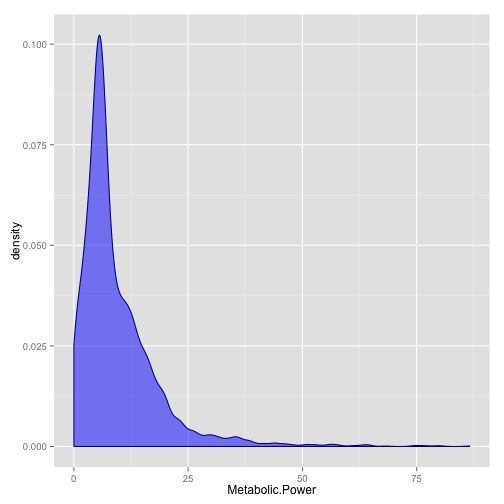As can be seen from the plot, most of the scores are <25 W/kg (note that VO2max or MAP - Maximal Aerobic Power is usually associated with 20 W/kg – see this blog post ), although there is a certain data spread (min = 0 and max = 86.62). Some of it might be the measurement error ( those over 100 W/kg) [That's why we need validity and reliability studies]

Here is the box plot. Note the outliers.

``````# create boxplot that includes outliers
ggplot(Catapult.data, aes(y = Metabolic.Power)) + geom_boxplot(aes(x = 1), fill = "steelblue",
alpha = 0.4, outlier.colour = "blue", outlier.shape = 23, outlier.size = 1) +
stat_summary(aes(x = 1), fun.y = "mean", geom = "point", shape = 23, size = 3,
fill = "white") + labs(x = "")
``````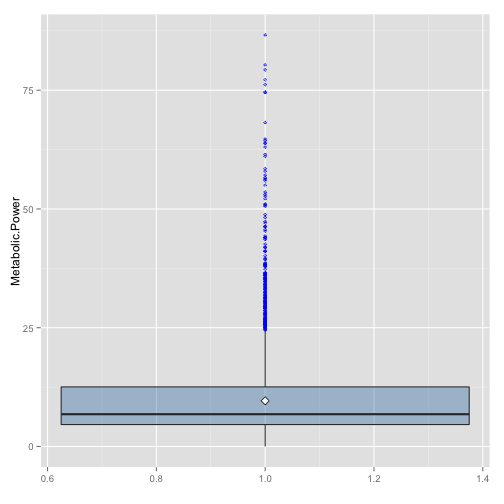I am not going to clean the outliers for now, but we usually should do it. For the sake of this example it is not necessary. Please note that outliers might be measurement error or really hard acceleration. This is why we need validity and reliability studies.

Here is the Metabolic Power over the duration of the sample (in this case SSG for 7 minutes)

``````# create boxplot that includes outliers
ggplot(Catapult.data, aes(y = Metabolic.Power, x = Time)) + geom_area(fill = "blue",
alpha = 0.4, color = "dark blue")
``````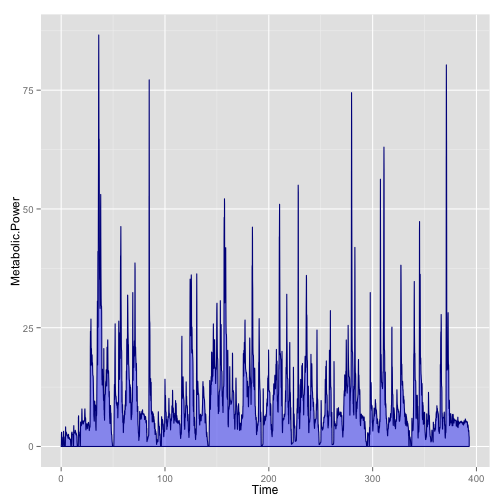Let's assume that critical power of the athlete is 13 W/kg. What we need to create is the difference between CP and MP and create a factor that tells wether we are in aerobic or anaerobic zone (according to CP/W' model, all work done over CP uses W' or anaerobic capacity - hence the call anaerobic, although metabolism is not that black or white)

``````Athlete.CP <- 13  # Critical Power (This is individualized)
Catapult.data\$CP <- Athlete.CP

# Calculate the difference between CP and MP
Catapult.data\$difference <- with(Catapult.data, (CP - Metabolic.Power))

# Code the phases into aerobic or anaerobic. When difference between MP and
# CP is <0 that's anaerobic work. If it is >= 0 that's aerobic work
Catapult.data\$Energy.Source <- factor(with(Catapult.data, ifelse(difference >=
0, "Aerobic", "Anaerobic")))
``````

Now we can compare Aerobic and Anaerobic work comparing the time spent in each (in this case number of samples)

``````ggplot(Catapult.data, aes(x = Energy.Source, fill = Energy.Source)) + geom_bar(stat = "bin",
alpha = 0.5, color = "black")
``````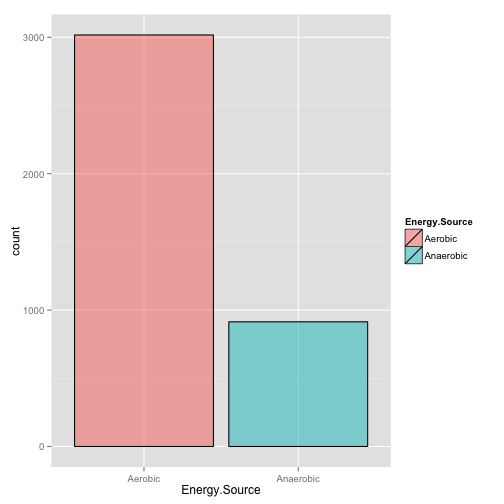Now it is the time to calculate spending and replenishing of W'. Please note that because MP and CP are expressed relatively to athletes BW then W' will also be expressed relatively to athlete's bodyweight. One assumption used here is that replenishment of W' is THREE time slower than W' depletion.

Let's assume that W' is 300 J/kg

One trick that needs to be covered is that W' needs to be capped to 300. In other way, if instant W' is 300 and MP < CP then the W' cannot increase. It can only decrease, and not below zero

``````Athlete.W.prime = 300
Catapult.data\$W.prime = Athlete.W.prime

for (i in seq(from = 2, to = length(Catapult.data\$Metabolic.Power))) {
w.change <- Catapult.data\$difference[i] * sampling

if (w.change > 0)
w.change <- w.change/3

new.w <- Catapult.data\$W.prime[i - 1] + w.change

if (new.w < Athlete.W.prime)
Catapult.data\$W.prime[i] <- new.w else Catapult.data\$W.prime[i] <- Athlete.W.prime
}
``````

On the following chart we can see what is happening with W' over time

``````ggplot(Catapult.data, aes(x = Time, y = W.prime)) + geom_path(color = "blue")
``````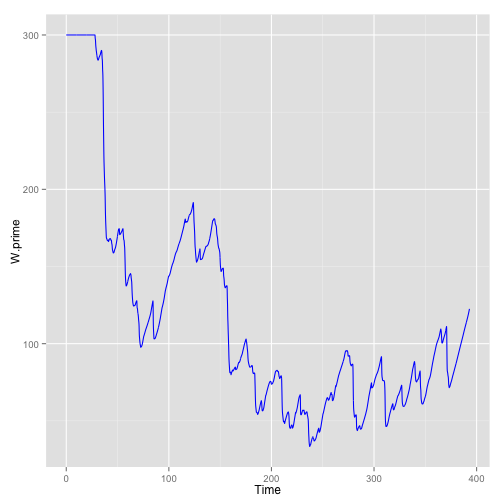Now we can write a function that calculates W' for a given data set and use it later in a nice simulation. We will add additional parameter W.start that defines the level of W' at the beginning of the sample. By the default this is same as athletes W'.

``````calculate.W.prime <- function(MetabolicPower, Time, Athlete.CP = 13, Athlete.W = 300,
Start.W = Athlete.W, replanishment.ratio = 1/3) {

# Create vectors and everything else needed for calculus
data.length <- length(MetabolicPower)
W.prime <- rep(Start.W, data.length)
dt <- c(NA, diff(Time))
MP.difference <- Athlete.CP - MetabolicPower

# Loop through elements and calculate W'
for (i in seq(from = 2, to = data.length)) {
w.change <- MP.difference[i] * dt[i]  # Calculate change in W

if (w.change > 0)
w.change <- w.change * replanishment.ratio  # Adjust ratio

new.w <- W.prime[i - 1] + w.change  # Calculate new W

# Check that we don't go over Athlete.W.prime
if (new.w < Athlete.W.prime)
W.prime[i] <- new.w else W.prime[i] <- Athlete.W.prime

# Check that we don't go below zero or exhaustion
if (new.w < 0)
W.prime[i] <- 0
}

return(W.prime)
}
``````

### Simulation

Now when we have function to easily calculate W' from data let's make a small simulation. Using the existing data from Catapult.data data.frame, let's assume that we have four players with different levels of CP, but the same levels of W'. In other words guys that differ in their aerobic fitness. What we want to see is how the same external work influences their W' levels over the duration of SSG drill.

``````Simulated.W <- data.frame(Time = Catapult.data\$Time, MP = Catapult.data\$Metabolic.Power)

Simulated.W\$Very.Low.Aerobic = with(Simulated.W, calculate.W.prime(MP, Time,
Athlete.CP = 11, Athlete.W = 300))

Simulated.W\$Low.Aerobic = with(Simulated.W, calculate.W.prime(MP, Time, Athlete.CP = 12,
Athlete.W = 300))

Simulated.W\$Medium.Aerobic = with(Simulated.W, calculate.W.prime(MP, Time, Athlete.CP = 13,
Athlete.W = 300))

Simulated.W\$High.Aerobic = with(Simulated.W, calculate.W.prime(MP, Time, Athlete.CP = 14,
Athlete.W = 300))
``````

Let's plot the scores, but before that we need to convert wide data format to long data format that is used by gglplot2. We will use melt function from reshape2 package.

``````library(reshape2)

drawing.data <- melt(Simulated.W, id.vars = c("Time", "MP"), variable.name = "Group",
na.rm = FALSE, )

levels(drawing.data\$Group) <- c("Very Low Aerobic", "Low Aerobic", "Medium Aerobic",
"High Aerobic")

ggplot(drawing.data, aes(x = Time, y = value, color = Group)) + geom_path() +
labs(x = "Time", y = "W'", fill = "Aerobic Fitness") + guides(color = guide_legend(reverse = TRUE))
``````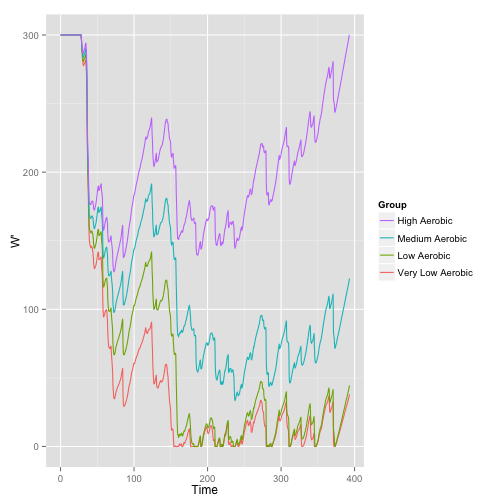As can be seen from the picture, athletes with lower CP will reach exhaustion, and won't be able to achieve such a work rate. Higher the CP, the less one spends W' for the sample supramaximal work and quicker he replenishes W' for work under CP.

Utilizing this approach might allow coaches real time fatigue monitoring of the players during the game or training.

### Validation

This is very interesting approach to fatigue estimation. But it needs to be validated. One approach might involve measuring levels of performance before, in the half time and at the end of the game and comparing this drop to W' drop. If this is correlated, then W' could be used for real-time estimation of fatigue.

One thing to note though - if we use very short burst type tests, like 10m sprint or vertical jump, we might not get the correlation with W'. One interesting study by Marcora et al. showed this. Researchers need to pay attention what they use for criterion measure. I believe that the drop in W' will mostly correlated with drop in a criterion test that has some duration like Wingate 30sec test on the bike, not necessarily drop in vertical jump height or power for example.

### Conclusion

I guess this represent one very interesting method with a lot of potential applications. This could easily be PhD thesis and a source of ideas for research. All I hope is that the potential users (companies, researchers, clubs) of this approach will remember to reference this blog and it's author.